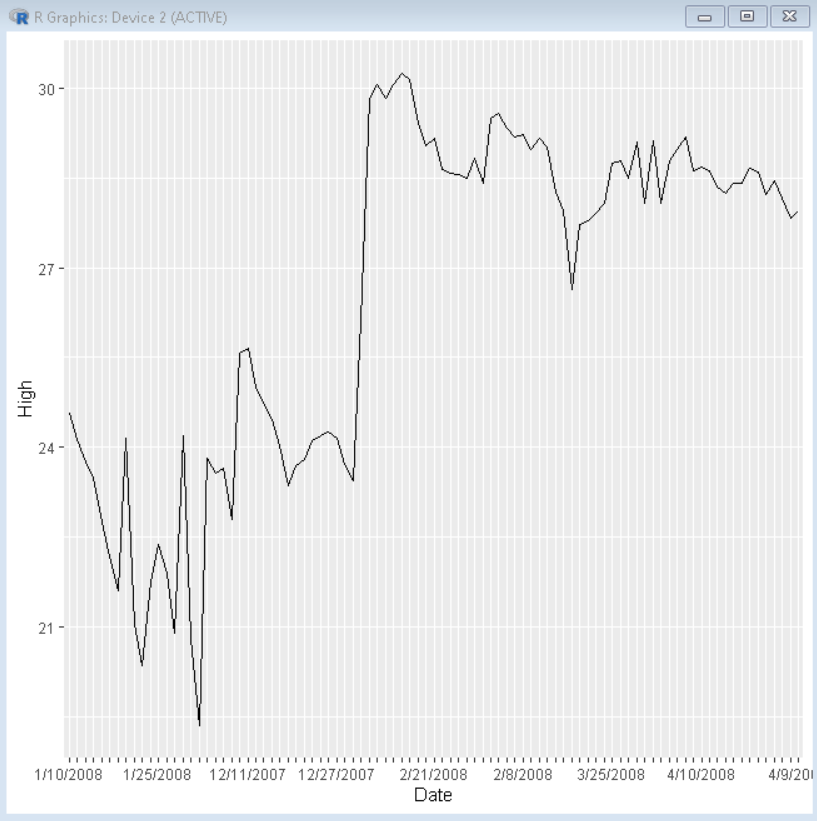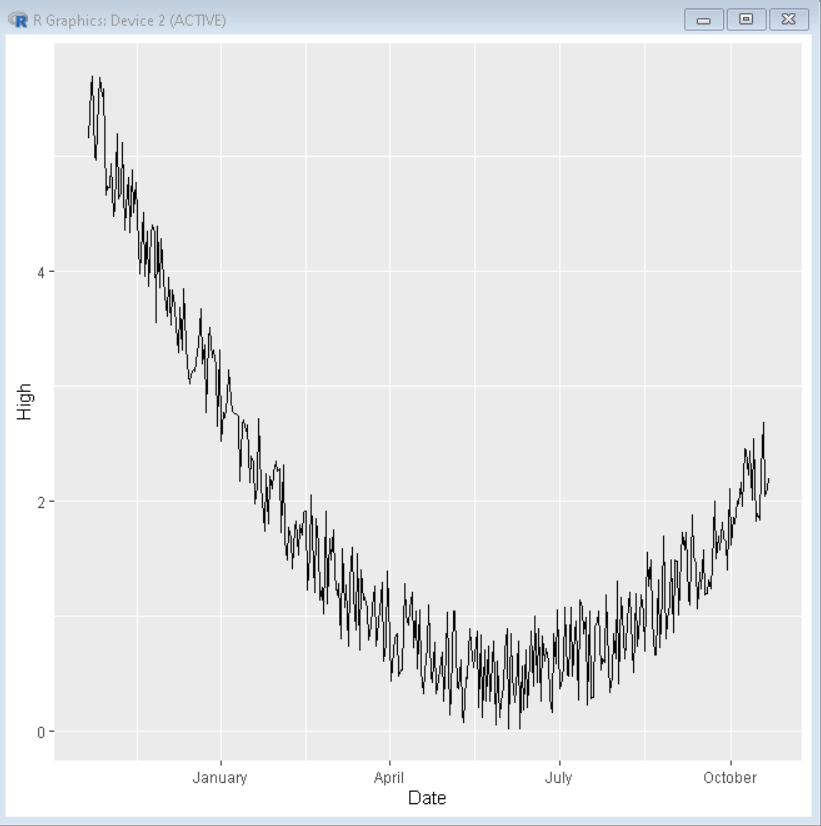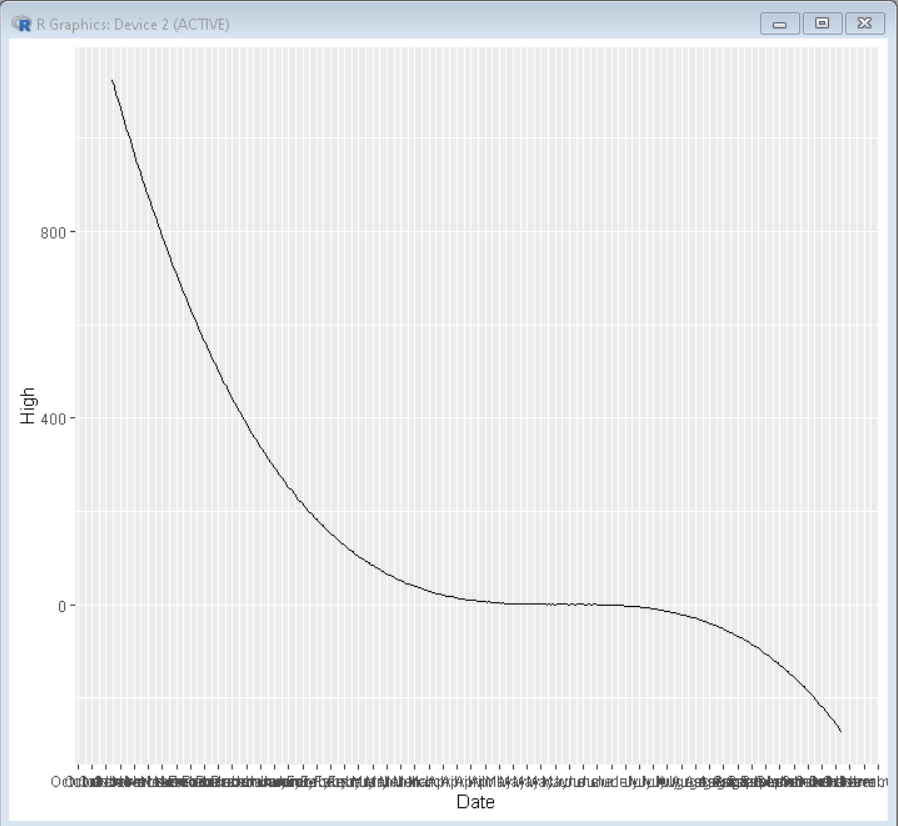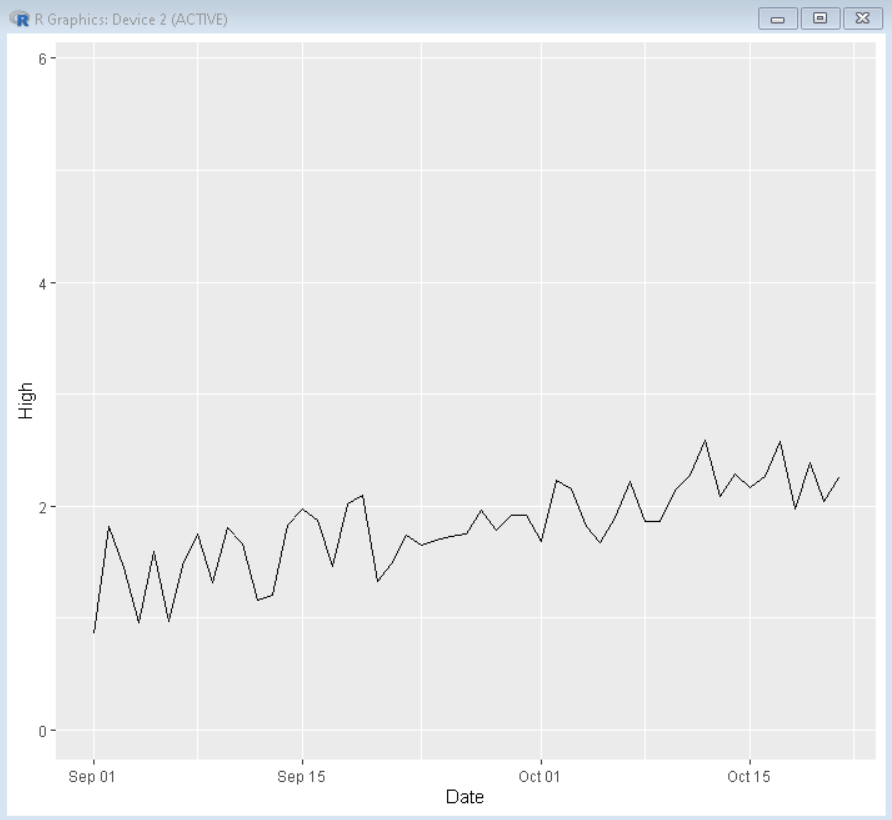GeeksforGeeks App
Open AppBrowser
Continue

# Time series visualization with ggplot2 in R

In this article, we will discuss time-series visualization with the ggplot2 package in the R programming Language.

A time series is the series of data points listed in the order timeline i.e. one of the axes in the form of dates, years, or months. A time series is a sequence of successive equal interval points in time. A time-series analysis consists of methods for analyzing time-series data in a way to retrieve some meaningful insight from data. Time-series data analysis is becoming very important in so many industries like financial industries, pharmaceuticals, social media companies, web service providers, research, and many more as it helps to predict future events based on trends of past data.

To better get in the skin of data we need some tools to visualize it. The R programming language provides a strong of tools in the ggplot2 package to visualize data. We can use the geom_line() function to visualize the time-series data using a line plot.

Syntax:

ggplot(dataframe , aes(x, y)) + geom_line()

Parameter:

• dataframe: determines the dataframe variable for plotting chart
• x: determines the time variable vector
• y: determines the data variable vector for time vector.

Example: A basic line chart depicting time series data.

Dataframe in use: here

## R

 `# load library ggplot2``library``(ggplot2)`` ` `# create timeseries dataframe``dataframe <- ``data.frame``(``read.csv``(``"sample2.csv"``))`` ` `# create plot``ggplot``(dataframe, ``aes``(x=Date, y=High, group = 1)) +``  ``geom_line``()+``# check.overlap avoids label overlapping``scale_x_discrete``(guide = ``guide_axis``(check.overlap = ``TRUE``))`

Output:## Data Label format

Since one of the axis data is in the form of date, we can format it in multiple forms of date. We can use the scale_x_date() function to choose the format displayed on the X-axis.

Syntax:

scale_x_date(date_labels = date-format-identifiers)

Here, date format identifiers are:

Example: A time series using data label identifier to display only month in long-form.

## R

 `# load library ggplot2``library``(ggplot2)`` ` `# create timeseries dataframe``dataframe <- ``data.frame``(``                      ``Date = ``as.Date``(``"2021-10-21"``) - 0:364,``                      ``High = ``runif``(365) + ``seq``(-140, 224)^2 / 10000)`` ` `# create plot``ggplot``(dataframe, ``aes``(x=Date, y=High, group = 1)) +``  ``geom_line``()+`` ` `# check.overlap avoids label overlapping``scale_x_discrete``(guide = ``guide_axis``(check.overlap = ``TRUE``))+`` ` `# change month format to long-form month``scale_x_date``(date_labels = ``"%B"``)`

Output:## Breaks and Minor breaks

We can create different breakpoints by using minor or major breaks in the plot using the date_breaks and date_minor_breaks argument of the scale_x_date() function of ggplot2.

Syntax:

scale_x_date(date_breaks, date_minor_breaks)

Example: A basic time series plot with manual date breaks and minor date breaks.

## R

 `# load library ggplot2``library``(ggplot2)`` ` `# create timeseries dataframe``dataframe <- ``data.frame``(``                      ``Date = ``as.Date``(``"2021-10-21"``) - 0:364,``                      ``High = ``runif``(365) + ``seq``(-140, 224)^3 / 10000)`` ` `# create plot``ggplot``(dataframe, ``aes``(x=Date, y=High, group = 1)) +``  ``geom_line``()+`` ` `# check.overlap avoids label overlapping``scale_x_discrete``(guide = ``guide_axis``(check.overlap = ``TRUE``))+`` ` `# change month format to long-form month``# date_breaks and date_minor_breaks for axis break``scale_x_date``(date_labels = ``"%B"``,date_breaks = ``"1 week"``)`

Output:## Limit Axis Data

While dealing with large datasets, we might need to focus on a small time frame. To do so we use the limit option of the scale_x_date() function to select a time frame in the data.

Syntax:

scale_x_date(limit)

Example: A plot with limited data from October 2021 to July 2021.

## R

 `# load library ggplot2``library``(ggplot2)`` ` `# create timeseries dataframe``dataframe <- ``data.frame``(``                      ``Date = ``as.Date``(``"2021-10-21"``) - 0:364,``                      ``High = ``runif``(365) + ``seq``(-140, 224)^2 / 10000)`` ` `# create plot``ggplot``(dataframe, ``aes``(x=Date, y=High, group = 1)) +``  ``geom_line``()+`` ` `# check.overlap avoids label overlapping``scale_x_discrete``(guide = ``guide_axis``(check.overlap = ``TRUE``))+`` ` `# limit axis data``scale_x_date``(limit=``c``(``as.Date``(``"2021-09-01"``),``as.Date``(``"2021-10-21"``)))`

Output:My Personal Notes arrow_drop_up